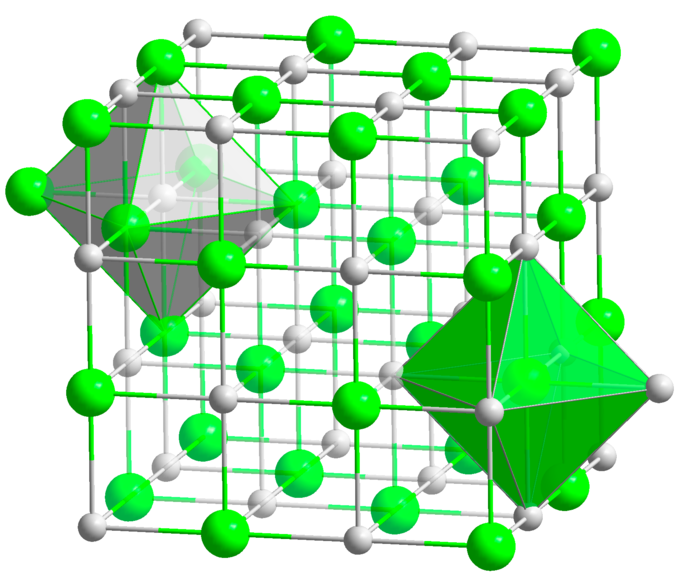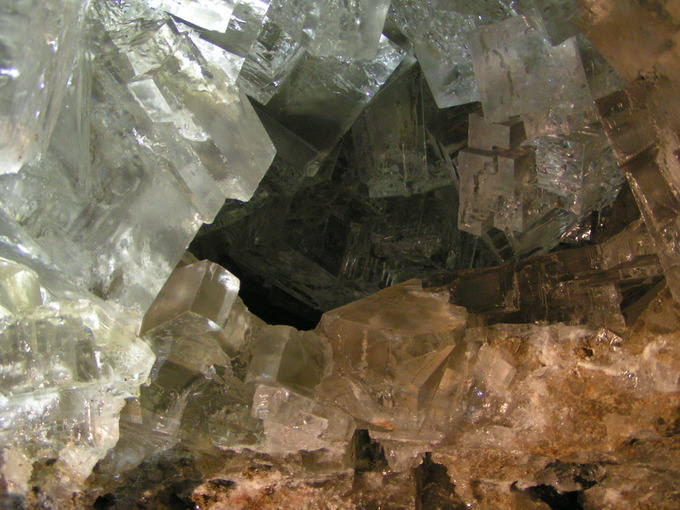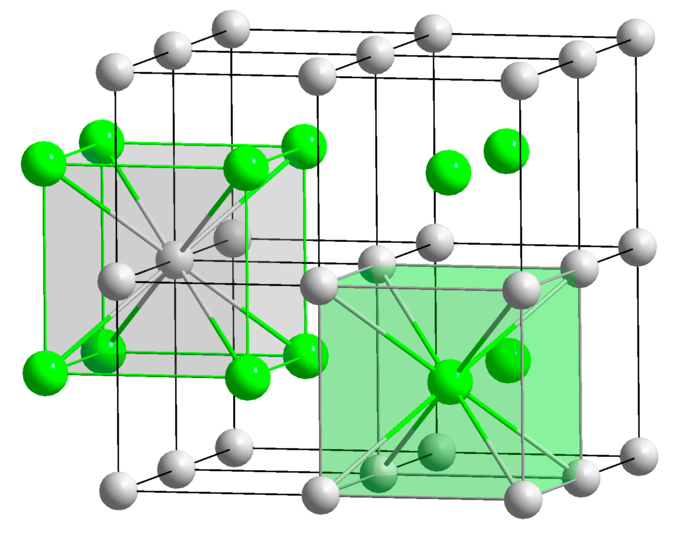## Ionic Crystals

#### Learning Objective

• Describe how ions form ionic crystals.

#### Key Points

• Ions bound together by electrostatic attraction form ionic crystals. Their arrangement varies depending on the ions’ sizes or the radius ratio (the ratio of the radii of the positive to the negative ion). A simple cubic crystal lattice has ions equally spaced in 3D at 90° angles.
• Stability of ionic solids depends on lattice energy, which is released in the form of heat when two ions are brought together to form a solid. Lattice energy is the sum of all the interactions within the crystal.
• The properties of ionic crystals reflect the strong interactions that exist between the ions. They are very poor conductors of electricity, have strong absorption of infrared radiation, and are easily cleaved. These solids tend to be quite hard and have high melting points.

#### Terms

• ionic crystalA class of crystal consisting of a lattice of ions held together by electrostatic interactions; they exhibit strong absorption of infrared radiation and have planes along which they cleave easily.
• crystal latticeA regular three-dimensional geometric arrangement of atoms, molecules, or ions in a crystal.
• lattice energyThe energy required to separate the ions of an ionic solid (especially a crystal) to an infinite distance apart.

## The Crystalline Form of Ionic Compounds

An ionic crystal consists of ions bound together by electrostatic attraction. The arrangement of ions in a regular, geometric structure is called a crystal lattice. Examples of such crystals are the alkali halides, which include:

• potassium fluoride (KF)
• potassium chloride (KCl)
• potassium bromide (KBr)
• potassium iodide (KI)
• sodium fluoride (NaF)
• other combinations of sodium, cesium, rubidium, or lithium ions with fluoride, bromide, chloride or iodide ions

These solids tend to be quite hard and have high melting points, reflecting the strong forces between oppositely-charged ions. The exact arrangement of ions in a lattice varies according to the size of the ions in the crystal.

## A Case Study: NaCl

The properties of NaCl reflect the strong interactions that exist between the ions. It is a good conductor of electricity when molten (melted state), but very poor in the solid state. When melted, the mobile ions carry charge through the liquid. NaCl crystals are characterized by strong absorption of infrared (IR) radiation, and have planes along which they cleave easily. Structurally, each ion in sodium chloride is surrounded by six neighboring ions of opposite charge. The resulting crystal lattice is of a type known as “simple cubic,” meaning that the lattice points are equally spaced in all three dimensions and all cell angles are 90°.NaCl crystal structureSpheres represent the Na+ and Cl- ions. Each ion is surrounded by six other ions of opposite charge, therefore NaCl is described as having (6,6) coordination.

How can one sodium ion surrounded by six chloride ions (or vice versa) be consistent with the simplest (empirical) formula NaCl? The answer is that each of those six chloride ions sits at the center of its own octahedron, whose vertices are defined by six neighboring sodium ions. This might seem to correspond to Na6Cl6, but note that the central sodium ion shown in the diagram can claim only a one-sixth share of each of its chloride ion neighbors. Therefore, the formula NaCl is not just the simplest formula, but correctly reflects the 1:1 stoichiometry of the compound. As in all ionic structures, there are no distinguishable “molecular” units that correspond to the NaCl simplest formula. Sodium chloride, like virtually all salts, is a more energetically favored configuration of sodium and chlorine than the elements individually.HaliteHalite, or rock salt, is the mineral form of sodium chloride. Halite forms cubic crystals. It occurs in evaporite minerals that result from the drying up of enclosed lakes and seas. This picture was taken in Wieliczka, Poland, one of the world’s oldest salt mines.

## Energy of Formation of Ionic Salts

Since ionic salts have a lower energetic configuration than their individual elements, reactions forming ionic solids tend to release energy. For example, when sodium and chlorine react to form sodium chloride:

Na(s) + ½Cl2(g) → NaCl(s) + 404 kJ

The release of 404 kJ of energy shows that the formation of solid sodium chloride is exothermic. Due to the Second Law of Thermodynamics, the released energy spreads out into the environment and is therefore unavailable to drive the reverse reaction. This irreversibility is the main reason that sodium chloride is more stable than its component elements.

## Lattice Energy

When sodium and chloride ions react to form NaCl, 787 kJ/mol of energy is released:

Na+(g) +Cl(g) → NaCl(s) + 787 kJ

This large magnitude arises from the strength of the coulombic force between ions of opposite charge. This energy is one definition of lattice energy: the energy released when an ionic solid is formed from gaseous ions binding together. Note that the actual value of enthalpy change (ΔHo) is negative (- 787 kJ/mol).

The exothermicity of such reactions results in the stability of ionic solids. That is, energy is needed to break apart the ionic solid into its constituent elements. (This is not to be confused with dissociation of a compound’s ions in solution. That is a different process.) This endothermic reaction gives rise to the other definition of lattice energy: the energy that must be expended to break up an ionic solid into gaseous ions.

Lattice energy, while due mainly to coulombic attraction between each ion and its nearest neighbors (six in the case of NaCl) is really the sum of all the interactions within the crystal. Lattice energies cannot be measured directly, but they can be estimated from the energies of other processes.

## Close-packed Structures

The most energetically stable arrangement of solids made up of identical molecular units are generally those in which there is a minimum of empty space. These are known as close-packed structures, and there are several kinds of them.

In ionic solids of even the simplest 1:1 stoichiometry, the positive and negative ions usually differ so much in size that packing is often much less efficient. This may cause the solid to assume lattice geometries that differ from the one illustrated above for NaCl.

Consider the structure of cesium chloride, CsCl. The radius of the Cs+ ion is 168 pm (compared to 98 pm for Na+), and cannot possibly fit into the octahedral hole of a simple cubic lattice of chloride ions (181 pm ionic radius). The CsCl lattice therefore assumes a different arrangement.CsCl structureIn CsCl, metal ions are shifted into the center of each cubic element of the Cl–-ion lattice. Each cesium ion has eight nearest-neighbor chloride ions, while each chloride ion is also surrounded by eight cesium ions in (8,8) coordination.

The two kinds of lattice arrangements exemplified by NaCl and CsCl are found in a large number of other 1:1 ionic solids, and these names are used generically to describe the structures of these other compounds. There are many other fundamental lattice arrangements (not all cubic), but the two described here are sufficient to illustrate the point that the radius ratio (the ratio of the radii of the positive to the negative ion) plays an important role in the structures of simple ionic solids.